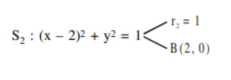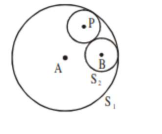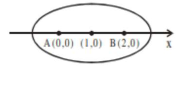# Let S1Question:

Let $S_{1}: x^{2}+y^{2}=9$ and $S_{2}:(x-2)^{2}+y^{2}=1$ Then the locus of center of a variable circle $S$ which touches $S_{1}$ internally and $S_{2}$ externally always passes through the points:

1. (1) $(0, \pm \sqrt{3})$

2. (2) $\left(\frac{1}{2}, \pm \frac{\sqrt{5}}{2}\right)$

3. (3) $\left(2, \pm \frac{3}{2}\right)$

4. (4) $(1, \mathrm{a} 2)$

Correct Option: 3,

Solution:$\because \mathrm{c}_{1} \mathrm{c}_{2}=\mathrm{r}_{1}-\mathrm{r}_{2}$$\therefore$ given circle are touching internally

Let a veriable circle with centre $\mathrm{P}$ and radius

r

$\Rightarrow \mathrm{PA}=r_{1}-r$ and $\mathrm{PB}=\mathrm{r}_{2}+\mathrm{r}$

$\Rightarrow \mathrm{PA}+\mathrm{PB}=\mathrm{r}_{1}+\mathrm{r}_{2}$

$\Rightarrow \mathrm{PA}+\mathrm{PB}=4(>\mathrm{AB})$

$\Rightarrow$ Locus of $P$ is an ellipse with foci at $A(0,0)$ and $B(2,0)$ and length of major axis is $2 \mathrm{a}=4$,

$e=\frac{1}{2}$

$\Rightarrow$ centre is at $(1,0)$ and $b^{2}=a^{2}\left(1-e^{2}\right)=3$

if $x$-ellipsewhich is satisfied by $\left(2, \pm \frac{3}{2}\right)$